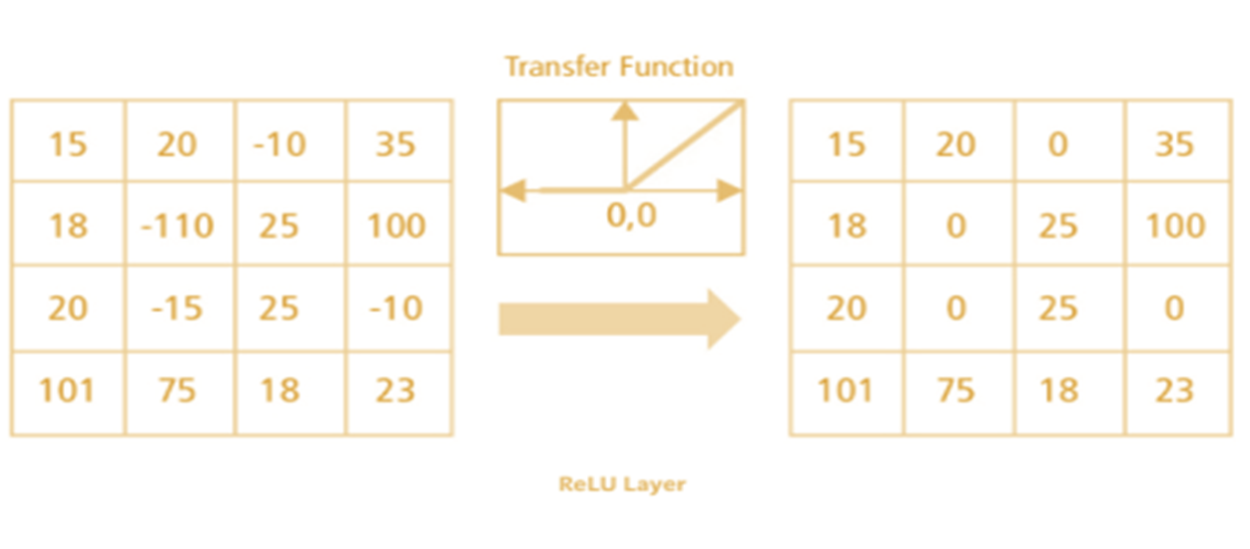Related Tags

general
community creator

# What is a ReLU layer?

A Rectified Linear Unit(ReLU) is a non-linear activation function that performs on multi-layer neural networks. (e.g., f(x) = max(0,x) where x = input value).

## What happens in ReLU layer?

In this layer we remove every negative value from the filtered image and replace it with zero. This function only activates when the node input is above a certain quantity. So, when the input is below zero the output is zero. However, when the input rises above a certain threshold it has linear relationship with the dependent variable. This means that it is able to accelerate the speed of a training data set in a deep neural network that is faster than other activation functions – this is done to avoid summing up with zero.

### A ReLU layer:

• performs element-wise operations.
• has an output that is a rectified feature map.

## Examples of ReLU

x f(x)=x F(X)
-110 f(-110)=0 0
-15 f(-15)=0 0
15 f(15)=15 15
25 f(25)=25 25RELATED TAGS

general
community creator

CONTRIBUTOR